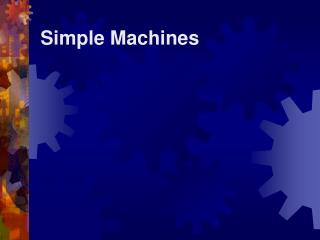# Simple Machines - PowerPoint PPT PresentationDownload PresentationSimple Machines

Simple MachinesDownload Presentation## Simple Machines

- - - - - - - - - - - - - - - - - - - - - - - - - - - E N D - - - - - - - - - - - - - - - - - - - - - - - - - - -
##### Presentation Transcript

1. Simple Machines

2. What is a Simple Machine? • A simple machine has few or no moving parts. • Simple machines make work easier

3. Wheels and Axles • The wheel and axle are a simple machine • Gears are a form of wheels and axles

4. Pulleys • Pulley are wheels and axles with a groove around the outside • A pulley needs a rope, chain or belt around the groove to make it do work

5. Inclined Planes • An inclined plane is a flat surface that is higher on one end • Inclined planes make the work of moving things easier

6. Wedges • Two inclined planes joined back to back. • Wedges are used to split things.

7. Screws • A screw is an inclined plane wrapped around a shaft or cylinder. • The inclined plane allows the screw to move itself when rotated.

8. Levers-First Class • In a first class lever the fulcrum is in the middle and the load and effort is on either side • Think of a see-saw

9. Levers-Second Class • In a second class lever the fulcrum is at the end, with the load in the middle • Think of a wheelbarrow

10. Levers-Third Class • In a third class lever the fulcrum is again at the end, but the effort is in the middle • Think of a pair of tweezers

11. Simple Machines • Simple Machines can be put together in different ways to make complex machinery

12. Simple Machines- Mechanical Advantage • Mechanical advantage (MA) is the ratio of the force put into the machine (Effort Force) to the force the machine exerts on an object (Resistance Force). • MA = Fr / Fe • MA > 1 means that the machine makes work easier and increases the amount of force applied

13. A sample problem • A worker uses a pulley to lift a 45 kg object. If the mechanical advantage of the pulley is 5.2, what is the effort force exerted by the worker? MA = Fr / Fe 5.2 = 441 N / Fe Effort Force = ??? Mass = 45 kg Resistance Force = 45 * 9.8 = 441 N Effort Force = 85 N

14. A sample problem – You try it • A worker uses a lever to lift an object with a mass of 3500 N. If the force applied by the operate was 1500 N, what is the mechanical advantage of the lever? MA = Fr / Fe MA = 3500 N / 1500 N Effort Force = 1500 N Resistance Force = 3500 N MA = 2.33

15. Simple Machines – Ideal Mechanical Advantage • Ideal Mechanical advantage is the ratio of distance moved by the person (de ) to the distance the machine moved the object (dr ). • IMA = de / dr LOOK AT PAGE 266 Figure 10-9

16. A Sample Problem- You Try it • A worker testing a multiple pulley system to estimate the heaviest object the system could move. When the worker moves the rope 1.5 m, the object moves 0.25 m. What is the IMA of the system? IMA = de / dr

17. Simple Machines - efficiency • Efficiency is the ratio of work output (Wo) to work input (Wi ) multiplied by 100. • Efficiency = e = (Wo / Wi ) *100 • Another way to calculate it using mechanical and ideal mechanical advantage • Efficiency = e = (MA / IMA) * 100

18. A sample problem • A man exerts a force of 310 N on a lever to raise a crate with a mass of 910 kg. If the efficiency of the lever is 78%, what is the lever’s IMA? • e = (MA / IMA) * 100 IMA = de / dr MA = Fr / Fe Effort Force = 310 N Mass = 910 kg Resistance Force = 910 * 9.8 = 8910 N • e = (MA / IMA) * 100 • 78 = (28.7 / IMA) *100 • IMA = 36.8 MA = 8910 / 310 MA = 28.7

19. A sample problem – You Try It • A man exerts a force of 650 N on a lever to raise a crate with a weight of 1000 N. If the efficiency of the lever is 55%, what is the lever’s IMA? • e = (MA / IMA) * 100 IMA = de / dr MA = Fr / Fe Effort Force = 650 N Resistance Force = 1000 N • e = (MA / IMA) * 100 • 55 = (1.53 / IMA) *100 • IMA = 2.8 MA = 1000 / 650 MA = 1.53

20. Homework • Page 280 • Problems: 79-84